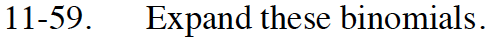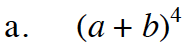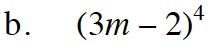Home > INT3 > Chapter 11 > Lesson 11.2.1 > Problem11-59

11-59.
1. Expand these binomials. Homework Help ✎

1. (a + b)4

2. (3m – 2)4For help with this problem, refer to problem 11-10 in Lesson 11.1.1.Use the results of part (a). Let a = 3m and b = −2. Simplify.

$81m^4 -216m^3 +216m^2 -96m+16$